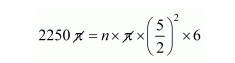# A hemispherical bowl of internal radius 15 cm contains a liquid.Question:

A hemispherical bowl of internal radius 15 cm contains a liquid. The liquid is to be filled into cylindrical-shaped bottles of diameter 5 cm and height 6 cm. How many bottles are necessary to empty the bowl?

Solution:

Internal radius of hemispherical bowl = 15 cm

The volume of bowl $=\frac{2}{3} \pi r^{3}=$ volume of liquid $=\frac{2}{3} \pi(15)^{3}$

Volume of liquid $=2250 \pi \mathrm{cm}^{3}$

Since, the liquid filled into the cylindrical shaped bottles of radius $\frac{5}{2} \mathrm{~cm}$ and height $6 \mathrm{~cm}$.

Let n be the no. of bottles.

Therefore,

The volume of liquid = n × volume of cylindrical shaped bottle$2250=n \times \frac{25}{4} \times 6$

$n=\frac{2250 \times 4}{25 \times 6}$

$=60$

$n=60$

Hence, the no. of bottles = 60